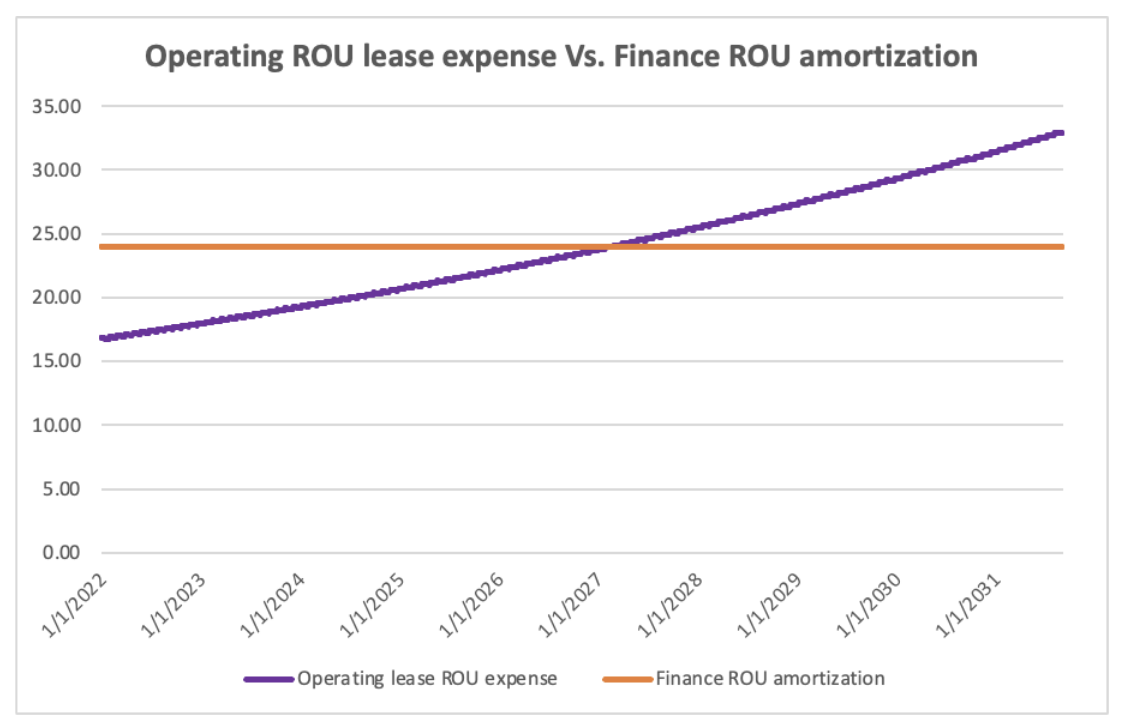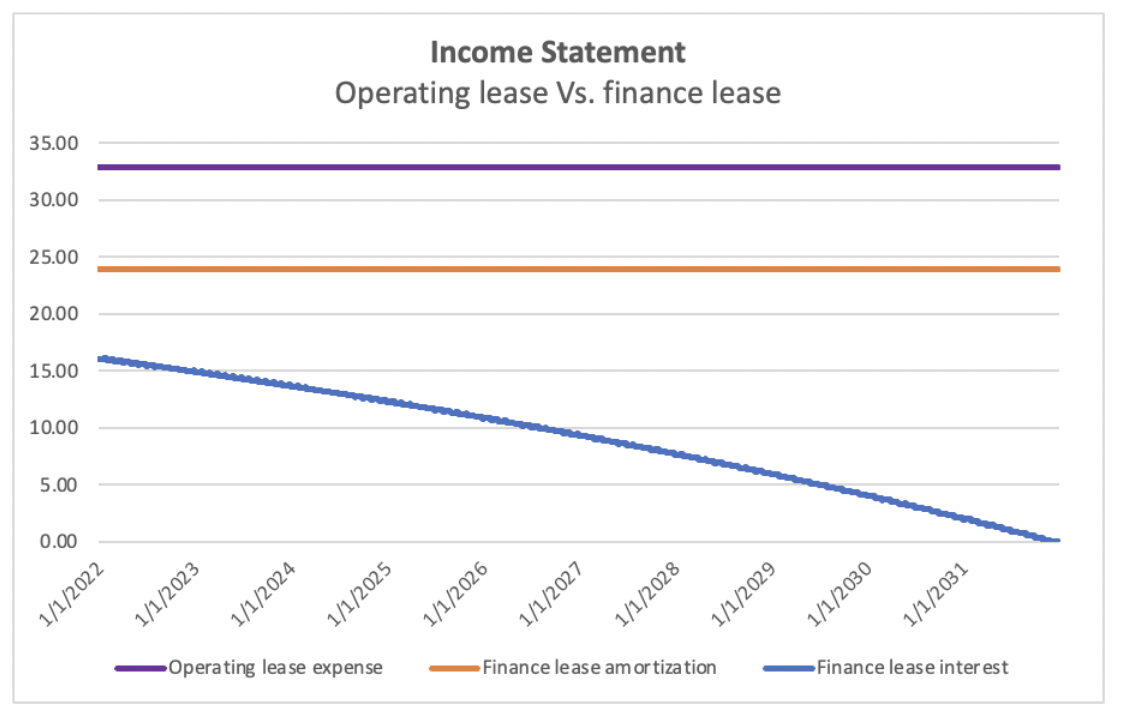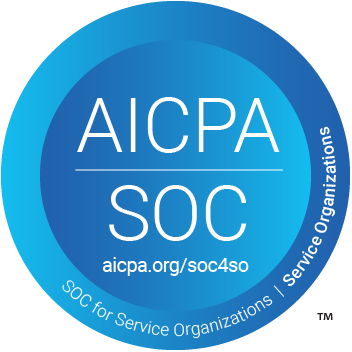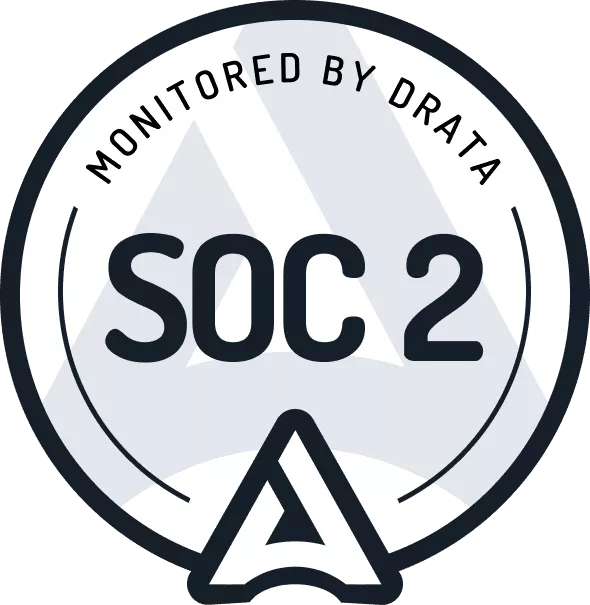# How to Calculate the Right of Use Asset Amortization and Lease Expense Under ASC 842

Lucas Russell 2022-08-12

## What is the Right of Use Asset?

The right of use asset is what a company recognizes on the balance sheet, representing the right to use the leased asset.

Under ASC 842, regardless of the classification of the lease, operating, or finance, a company must recognize a right of use asset for the majority of leases. This is a big difference from ASC 840!

Given the lease is for a stipulated period, the right of use asset must go to zero when the lessee no longer has control over the leased asset. This principle is no different from the amortization of an intangible asset.

## What is the Lease Expense of the Right of Use Asset for an Operating Lease?

The calculation of getting the ROU asset to zero differs depending on the lease classification.

For an operating lease, the lease expense will reduce the ROU asset to zero. There is a specific methodology for calculating this expense, which this article will cover in detail.

## Is the Calculation of Amortization for a Finance Lease Right of Use Asset Different?

Reducing the ROU asset value as the leased asset is used for a finance lease is not classified as a lease expense. Instead, it's an amortization expense.

The lessee can use any systematic approach to calculate the amortization amount. A straight line calculation is the easiest way to do this. The calculation of the finance lease is far more straightforward than an operating lease.

Calculating the amortization and lease expense is not the only difference between accounting for an operating and finance lease. For more differences, refer to this article:

The calculation of the amortization of a finance lease is very straightforward if you adopt the straight-line methodology. Right of use asset value is divided by the useful life, which is the amortization expense recognized yearly.

The following graph clearly shows the difference between the two methodologies when comparing finance and operating lease with identical lease terms:The finance lease ROU amortization is intuitive to understand as it is the same straight line expense each year, from January 1, 2022, to December 31, 2031.

The ROU operating lease expense increases year on year. This is because the operating lease has two expense items, the calculation of the interest related to the lease liability and the ROU asset. The following graph should help illustrate this point:The above diagram highlights that:

• Purple line: The total operating expense is a straight line expense.
• Orange line: The amortization of the finance lease ROU asset is a straight line expense.
• Blue line: The interest expense on the finance lease liability decreases over time.

Comparing the two graphs helps show why the operating lease ROU expense increases over time. As mentioned, the total operating lease expense comprises the operating ROU expense plus the lease liability interest expense. The ROU expense rises over time because the interest expense on the lease liability balance decreases.

Hint!

It’s important to highlight that a lessee recognizes a lease liability in conjunction with the right of use asset. The lease liability represents the future payments related to the lease. The lease liability balance will also be amortized to zero. From this balance, the right of use asset is calculated. For more information on the lease liability and how to calculate it refer to ASC 842 guide here

For further information on how to calculate the lease liability expense, refer to the following articles:

## How to Calculate the Right of Use Asset Expense

There are two ways to manually calculate the lease expense of the right of use asset. Or you could stop reading this article and let Cradle’s software do it for you, error-free ;)

The two methods prescribed are called different things depending on the accounting firm. For example, KPMG refers to the methodologies as method 1 and method 2. At the same time, Deloitte refers to the calculation methodologies as Approach A ROU Asset Balance Derived From Lease Liability and Approach B: Application of the “Plug” Approach.

We’ll now go through each available method.

### Method 1 - ROU Asset Balance Derived from Lease LiabilityThe carrying amount of the ROU asset is derived from the carrying amount of the lease liability at the end of each reporting period – i.e., a lessee measures the ROU asset as follows. [842-20-35-3(b)]

The benefit of choosing this method is it will allow entities to adopt the new lease accounting standard without significant changes to systems or processes. However, a lessee should only use method 1 if the entity intends not to recognize the ROU assets until it closes its books for the financial reporting period.

That’s because rather than tracking the ROU asset in its general ledger, at each reporting date the lessee creates a journal entry to:

• Calculate the current value of the lease liability journal that balance (Credit)
• Reverse any accrual-based operating lease accounting balances such as prepaid or accrued rent (debit/credit)
• Debit the ROU asset for the balance amount.

Method 1 is a back-of-the-envelope calculation of the lease expense of the right of use asset. This methodology will suit a company with a few straightforward leases.

Method 1 is a very manual approach and is hard to calculate when applied to an extensive portfolio of leases, especially in the context of the more complex circumstances that will arise under the guidance in Topic 842 – e.g. modifications, remeasurements, impairments, and foreign currency translation adjustments.

Based on this, we do not recommend adopting method 1, especially given method 2 does not result in a higher investment of time and is more accurate. Because of this, we will not provide an example of this methodology.

### Method 2 - Straight Line Lease Expense Less Liability InterestMethod 2 is the most systematic approach to calculating the lease expense for the right of use asset. It follows a similar logic of calculating amortization expense and deducting that to get the carrying amount of the right of use asset. Like you would for a finance lease.

The steps involved in this method are:

1. Calculate the total lease expense, that’s the total known lease payments added up (a)
2. Divide that by the number of periods of the lease e.g., day or years (b)
3. That provides the straight line lease expense (a) / (b) = (c)
4. Subtract the liability interest for the straight line lease expense for the relevant period, and that will give you the lease expense incurred on the right of use asset.

By using Method 2, it is easiest to implement a calculation process for each lease. Most importantly, the lessee can track the values of the lease liability and right of use asset at any time. In addition to this, it is more likely to be effective for addressing the more complex lease scenarios that are likely to arise for many lessees. For example, extension to lease terms or increase in payments can be handled systematically.

If you are going to comply with ASC 842 manually, we would highly recommend adopting this method.

### Impairment of the Right of Use Asset

There is a caveat to the above. If a lessee impairs the right of use of an asset, it then adopts a straight line amortization approach identical to that of a finance lease. As a result, both method 1 and method 2 are no longer applicable. This is stated explicitly in paragraph 842-20-25-7 of the standard.

This accounting involved in doing this is:

1. Determine the carrying amount of the ROU asset post impairment
2. Divided that value by the earlier of the useful life, the ROU asset, or the lease term's end.

This methodology will be applied until the right of use asset is amortized to zero. Note this is applied on a lease-by-lease basis.

## How to Calculate the Amortization Expense for a Finance Lease

As mentioned previously, it’s straightforward! The lessee can use any systematic approach to calculate amortization. We’ll cover the most simple – a straight-line methodology.

The initial recognition value of the ROU asset is divided by the useful life. For example, the initial recognition value is \$10,000, and the useful life is five years. This results in an amortization expense of \$2,000 per year. A lot more straightforward than an operating lease.

## How to Calculate the Initial Recognition Value of the Right of Use Asset

When using Method B for an operating lease or have a finance lease, the lessee must calculate the initial value of the right of use asset. There are several inputs to consider when calculating the initial recognition value of the ROU asset:The ROU asset is be measured as the lease liability value adjusted if applicable by:

(1) accrued or prepaid rents (i.e., the aggregate difference between the cash payment and straight-line lease cost)

(2) remaining unamortized initial direct costs and lease incentives, and

(3) impairments of the ROU asset

Once we have calculated the starting balance of the right of use asset, the lessee can calculate the starting value of the right of use asset for an operating lease and then calculate the lease expense.

## Examples

With an operating lease, because the lease expense is intrinsically tied to the calculation of the lease liability, an example must also include the calculation of the lease liability.

For a detailed explanation of how to calculate the right of use asset lease expense, refer to the following article:

For a finance lease, the amortization is a lot simpler, but nonetheless you can refer to a detailed example here:

## Conclusion

The calculation of the ROU asset lease expense is not a straightforward exercise. This is where you may want to consider using Cradle, as we automate all of these calculations.

If you choose the manual route, the provided examples of calculations should help you tackle the requirements.

## FooterHere at Cradle, our mission is simple; it's at the foundation of everything that we do. We want to make accountants' lives easier by leveraging technology to free up their time to focus on running the business.

140 Yonge St.
Suite 200
Toronto, ON M5C 1X6

US
Suite #73591
Torrance, CA 90503
USA

### Legal